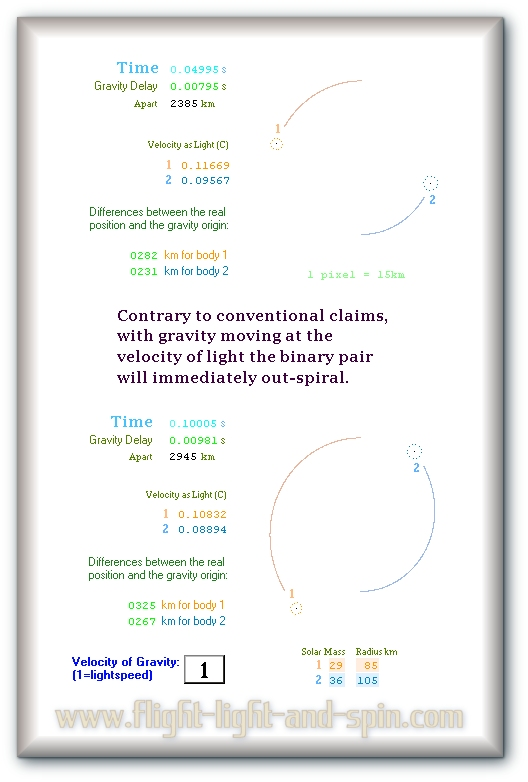The affect of gravity velocity on binary orbits (BOGVOS)

 1 2 3 4 5 6 7 8 9 10
 5 Each of the binary pair travels at a significant velocity when compared to one another (unlike a singular system). So when we take into account the force of gravity itself having velocity then we must realize that the origin of the pull of gravity cannot be at the same position as the body itself.In light of this, just using your imagination, try and figure out a rough idea of how the shape of the orbit changes with the gravity having velocity. .
 BOGVOS: Binary-Orbit-Gravity-Velocity-Out-Spiral.
 This needs to be explained with a much simpler approximation: At about 2500km apart, a binary pair of equal mass, (both 33 solar masses) travels at roughly one-tenth the velocity of light. So the delay in gravity causes a divergence between the gravity origin and the body itself of about one-tenth the distance between them. There is thus a gap of about 250 km between where the body actually is and where it was when it generated the gravity that eventually reaches the opposing body. Because the bodies travel at 1/10th the velocity of light, the gap between the body and the gravity origin is about 1/10 of the distance between the pair of bodies. That is a very big gap, proportionally speaking. And it is simple to verify the computation with simple arithmetic. The gap is demonstrated in the graphic above by the gap between the body and the orbit line (at the numbers 2 & 1). There are many conclusions that can be attained from this. Not the least of which is that the LIGO group have not solved the many-body-problem which is the engine driving the OGS11 computer models. In retrospect it is astonishing that nobody else seems to have realized that the pair will spiral outwards. .

 Next Page ->

^ Top of Page ^